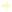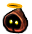View Single PostLodinn
 06.25.2020 , 02:44 AM | #17 Quote Quote: Originally Posted by Void_SingerProbably should have mentioned that calculation (95% ^ Attempts) only works for dominant odds over 50%, otherwise you have to calculate it as (1000% - [100% - non_dominant] ^ Attempts) which isn't very intuitive, but does give the right answer. I could only assume you actually meant 100% - [100% - non_dominant] ^ Attempts for success rate which is not only very intuitive, it's literally the same formula being used above. Get rid of the "dominant" and "non-dominant" notion altogether, it makes no difference at all because the calculation here is: 1) Find the probability of independently failing every time (since we're looking for 1 success only) - that's p_failure^attempts, or (1 - "chance to research the schematic")^deconstructions 2) Find the probability of this NOT happening = 1 - probability from the previous calculation. No matter if the success rate is 1% or 99.9% - the formula stays the same, the numbers are changing. For say 30 attempts that gives: At 95% failure chance the calculation would be 0.95^30=21.5% chance of not obtaining a single success in 30 trials At 5% failure chance it becomes 0.05^30=9.3e-40 chance of not obtaining a single success in 30 trials which, for all practical purposes, is a given success. That's just statistics 101, the part that is normally taught on like the very first lecture. Lterri | Estiuette | Shulmanu |Tenebrosa | Fabantou | Sneitha | Ton'itra | Lodinn | Flčchette | Tiponi | Pls'taunt | Gjalla/This'is'madness | Guédé | Cornifer TRE pugging all the way [IMP]